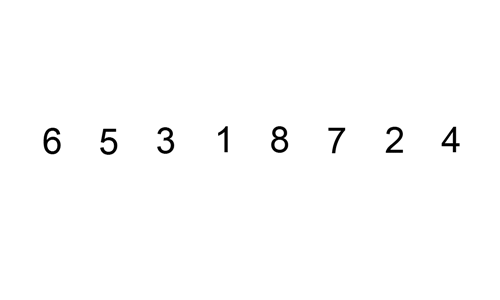# Insertion Sort - 插入排序

1. 從第一個元素開始，該元素可認為已排序
2. 取下一個元素，對已排序陣列從後往前掃描
3. 若從排序陣列中取出的元素大於新元素，則移至下一位置
4. 重複步驟3，直至找到已排序元素小於或等於新元素的位置
5. 插入新元素至該位置
6. 重複2~5

• 交換操作和陣列中導致的數量相同
• 比較次數>=倒置數量，<=倒置的數量加上陣列的大小減一
• 每次交換都改變了兩個順序顛倒的元素的位置，即減少了一對倒置，倒置數量為0時即完成排序。
• 每次交換對應著一次比較，且1到N-1之間的每個i都可能需要一次額外的記錄(a[i]未到達陣列左端時)
• 最壞情況下需要~$N^2/2$次比較和$~N^2/2$次交換，最好情況下需要$N-1$次比較和0次交換。
• 平均情況下需要~$N^2/4$次比較和~$N^2/4$次交換## Implementation

### Python

#!/usr/bin/env python

def insertionSort(alist):
for i, item_i in enumerate(alist):
print alist
index = i
while index > 0 and alist[index - 1] > item_i:
alist[index] = alist[index - 1]
index -= 1

alist[index] = item_i

return alist

unsorted_list = [6, 5, 3, 1, 8, 7, 2, 4]
print(insertionSort(unsorted_list))


### Java

public class Sort {
public static void main(String[] args) {
int unsortedArray[] = new int[]{6, 5, 3, 1, 8, 7, 2, 4};
insertionSort(unsortedArray);
System.out.println("After sort: ");
for (int item : unsortedArray) {
System.out.print(item + " ");
}
}

public static void insertionSort(int[] array) {
int len = array.length;
for (int i = 0; i < len; i++) {
int index = i, array_i = array[i];
while (index > 0 && array[index - 1] > array_i) {
array[index] = array[index - 1];
index -= 1;
}
array[index] = array_i;

/* print sort process */
for (int item : array) {
System.out.print(item + " ");
}
System.out.println();
}
}
}


template<typename T>
void insertion_sort(T arr[], int len) {
int i, j;
T temp;
for (int i = 1; i < len; i++) {
temp = arr[i];
for (int j = i - 1; j >= 0 && arr[j] > temp; j--) {
a[j + 1] = a[j];
}
arr[j + 1] = temp;
}
}


## 希爾排序 Shell sort

template<typename T>
void shell_sort(T arr[], int len) {
int gap, i, j;
T temp;
for (gap = len >> 1; gap > 0; gap >>= 1)
for (i = gap; i < len; i++) {
temp = arr[i];
for (j = i - gap; j >= 0 && arr[j] > temp; j -= gap)
arr[j + gap] = arr[j];
arr[j + gap] = temp;
}
}